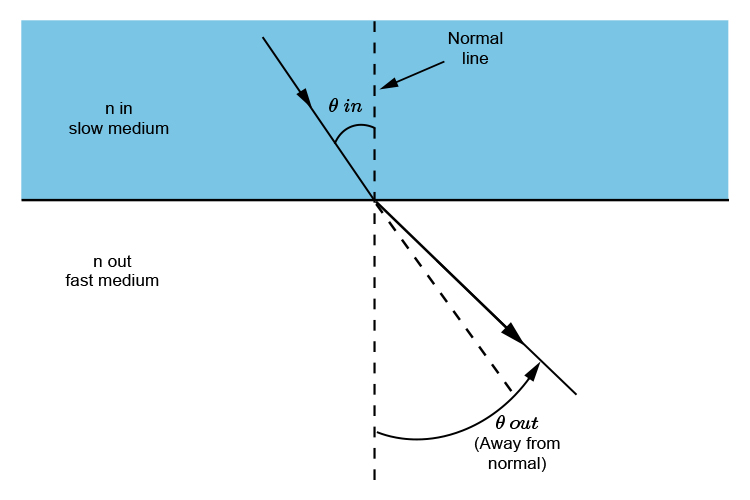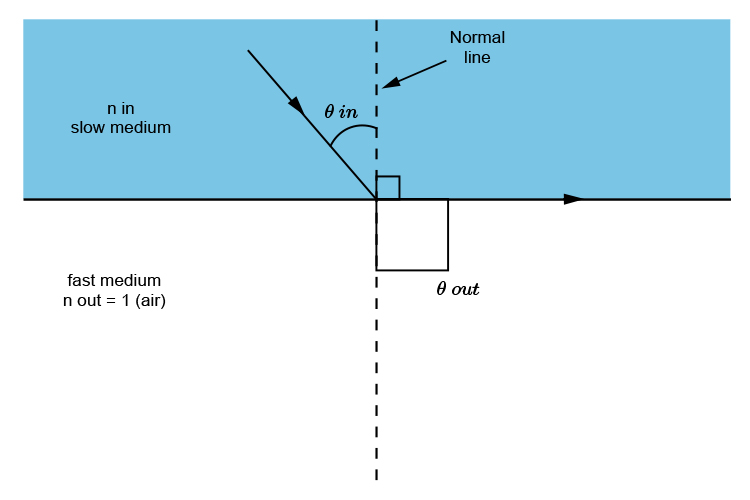# Working out the critical angle

The critical angle (where theta\ \o\u\t=90^circ) can be worked out for different media using the following process.

Use

sin\ \i\n\theta=1/(n(i\n))

becauseRemember FAST SOFA (slow in fast out away from the normal line)

and remember

(sin\ \i\n\theta)/(sin\ \o\u\t\theta)=(n\ \(o\u\t))/(n\ \(i\n))

but because theta\ \o\u\t is at 90^circ (sin90=1) and n\ \o\u\t is 1 (for air) . . .. . . the formula becomes

(sin\ \i\n\theta)/1=1/(n\ \(i\n))

and simplified

sin\ \i\n\theta=1/(n\ \(i\n))

## Water to air

(water n=1.3)

sin\ \i\n\theta=1/1.3=0.769

theta=sin^-1\0.769

theta=50.26~~50^circ

## Glass to air

(Glass n=1.5)

sin\ \i\n\theta=1/1.5=0.66dot 6

theta=sin^-1\0.66dot 6

theta=41.81~~42^circ

## Diamond to air

(Diamond n=2.417)

sin\ \i\n\theta=1/2.417=0.4137

theta=sin^-1\0.4137

theta=24.44~~24^circ

## Conclusion

The critical angle for

 Water to air ~~50^circ Glass to air ~~42^circ Diamond to air ~~24^circ